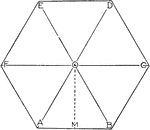### Area of Hexagon

Illustration showing how to find the area of a hexagon using the triangles that make it up.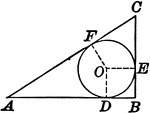### Circle Inscribed in a Right Triangle

Illustration showing the diameter of a circle inscribed in a right triangle is equal to the difference…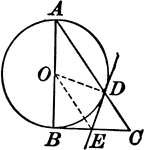### Circle With a Right Triangle

Illustration where one leg of a right triangle is the diameter of a circle. The tangent at the point…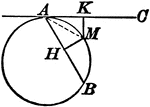### Circle With a Tangent Line and Chord

Illustration showing that from any point in the circumference of a circle, a chord and a tangent are…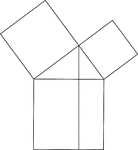### Euclid's Pythagorean Theorem Proof

Illustration used to prove the Pythagorean Theorem, according to Euclid. A perpendicular is drawn from…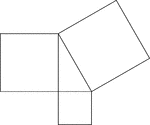### Geometric Pythagorean Theorem Proof

Illustration that can be used to prove the Pythagorean Theorem, the sum of the squares of the legs is…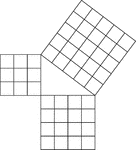### Geometric Pythagorean Theorem Proof

Illustration that can be used to prove the Pythagorean Theorem, the sum of the squares of the legs is…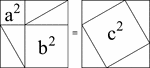### Pythagorean Theorem Proof by Rearrangement

A visual illustration used to prove the Pythagorean Theorem by rearrangement. When the 4 identical triangles…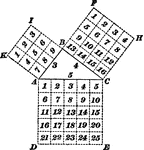### Right Triangle

"In any right triangle, the square described on the hypotenuse is equal to the sum of the squares described…### Steel Square

Illustration of steel square with hypotenuse drawn.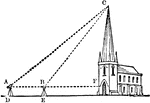### Triangle with Church

An illustration of a triangle comprised of a church and two lines. This illustration can be used to…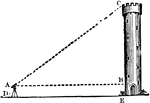### Triangle with Tower

An illustration of a triangle comprised of a tower and two lines. This illustration can be used to determine…### Triangle with Tree

An illustration of a triangle comprised of a tree and two lines. This is an example of a problem that…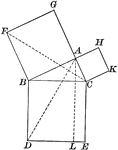### Right Triangle

Illustration of a right triangle used to show the Pythagorean Theorem (the square of the hypotenuse…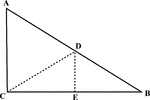### Right Triangle With Midpoint of Hypotenuse Drawn

Illustration of a right triangle with the midpoint of the hypotenuse drawn.